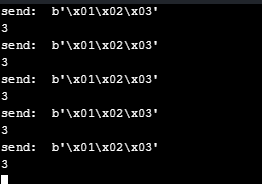lopy4 uart.write() problem

• My problem is that read from lopy4 uart will work but write will not work.

The pin is designated as G4, G15.
But it does not write.
The code is written as follows:

import time#uart = UART(1, baudrate=9600, pins=('G4', 'G15')) 1, 3uart = UART(1, 9600)                         # init with given baudrateuart.init(9600, bits=8, parity=None, stop=1, pins=('G4', 'G15')) # init with given parameterswhile True:
time.sleep(1)
send = bytes([1,2,3])
print('send: ', send)
b = uart.write(send)
print(b)

The results are as follows.I tried with two lopy4, but nothing was written.

In lopy4, G4 is tx and G15 is rx. So Arduino(mkrwan 1300) connected G4 to RX and G15 to TX.
(For reference, we first confirmed that the ping pong example works normally via two uarts.)

But Arduino did not receive a message from lopy4.

The following is summarized.

1. Data written in Arduino could be read in lopy.
2. The data written in Arduino was read from Arduino.
3. The data written in lopy4 could not be read by Arduino.

I was trying to write data to the ublox-based gps module via lopy4, but I'm having trouble because it does not work. I want to know if there is a problem I'm missing.

• @johand And so the code lacks an error message. Same with machine.Pin in general, which does not stop you on defining a Input only pin as output.

• In the pinout (https://docs.pycom.io/.gitbook/assets/lopy4-pinout.pdf) you can see that G4 (P14) is a read only pin. Try another pin.

Johan